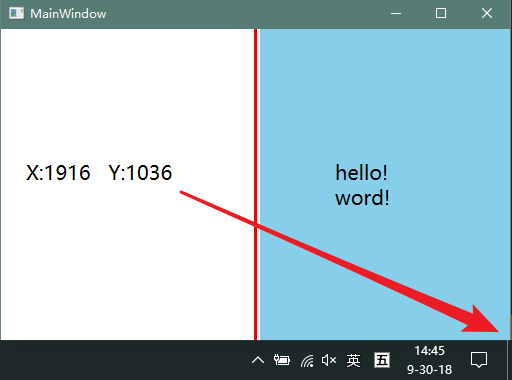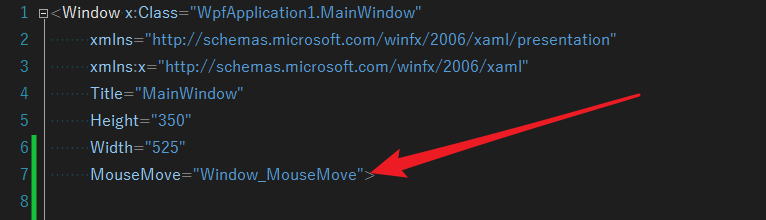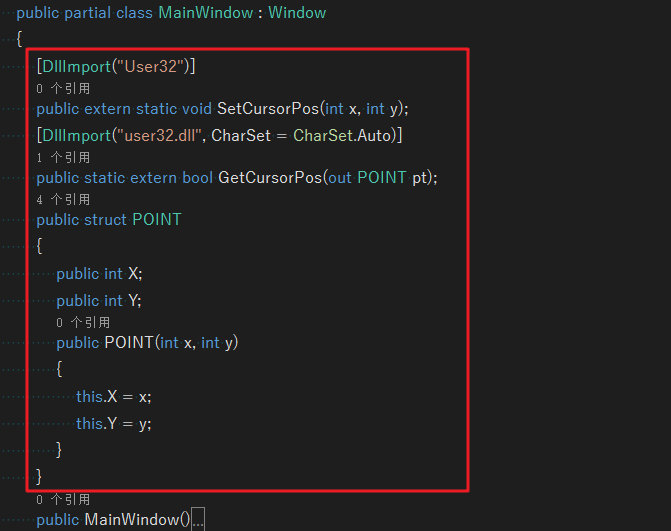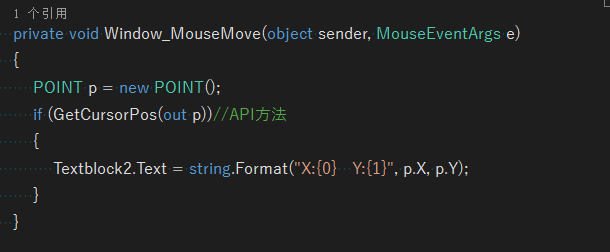VS编程，WPF中，获取鼠标相对于当前屏幕坐标的一种方法

效果2、为整个界面定义鼠标移动事件3、后台代码中增加如下代码：

        [DllImport("User32")]
public extern static void SetCursorPos(int x, int y);

[DllImport("user32.dll", CharSet = CharSet.Auto)]
public static extern bool GetCursorPos(out POINT pt);

public struct POINT
{
public int X;
public int Y;
public POINT(int x, int y)
{
this.X = x;
this.Y = y;
}
}3、在鼠标移动事件中，增加代码

             POINT p = new POINT();
if (GetCursorPos(out p))//API方法
{
Textblock2.Text = string.Format("X:{0}   Y:{1}", p.X, p.Y);
}